Next: Two-Dimensional Sources and Sinks Up: Two-Dimensional Incompressible Inviscid Flow Previous: Velocity Potentials and Stream

# Two-Dimensional Uniform Flow

Consider a steady two-dimensional flow pattern that is uniform: in other words, a pattern which is such that the fluid velocity is the same everywhere in the-plane. For instance, suppose that the common fluid velocity is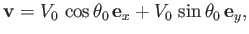(5.27)

which corresponds to flow at the uniform speed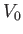in a fixed direction that subtends a (counter-clockwise) angle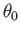with the-axis. It follows, from Equations (5.5) and (5.6), that the stream function for steady uniform flow takes the form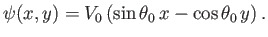(5.28)

When written in terms of cylindrical coordinates, this becomes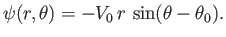(5.29)

Note, from Equation (5.28), that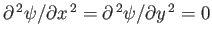. Thus, it follows from Equation (5.10) that uniform flow is irrotational. Hence, according to Section 4.15, such flow can also be derived from a velocity potential. In fact, it is easily demonstrated that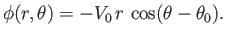(5.30)Next: Two-Dimensional Sources and Sinks Up: Two-Dimensional Incompressible Inviscid Flow Previous: Velocity Potentials and Stream
Richard Fitzpatrick 2016-03-31